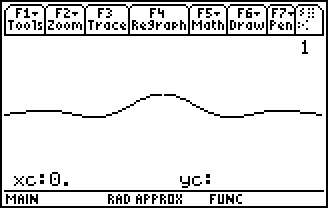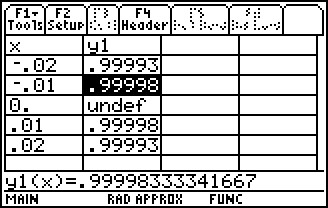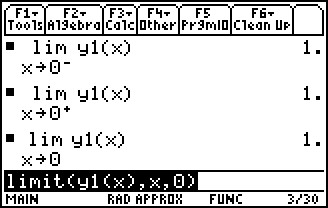# Activities

••• ##### Subject Area

• Math: Calculus: Limits of Functions

• ##### Author9-12

15 Minutes

• ##### Device
• TI-89 / TI-89 Titanium

## Limit of Sin(x)/x#### Activity Overview

In this activity, students will graph f(x)=sin(x)/x in order to visually determine the limit as x approaches zero. They will confirm the answer numerically by tracing left and right limit points to capture values in a spreadsheet.

#### Key Steps

•In this activity, students begin with an introduction the term indeterminate. Then they graph f (x) = sin(x)/x in order to quickly visually determine the limit as x approaches zero. They also explore the graph using Trace.

•Students set up the table and numerically investigate the values. In order to emphasize that for a limit to exist it must be approaching the same value from both sides, students will examine both sides of zero.

•Students use CAS to algebraically determine the limit. CAS connects the formal mathematical notation of limits to the visual representation examined earlier. They are also given practice problems.